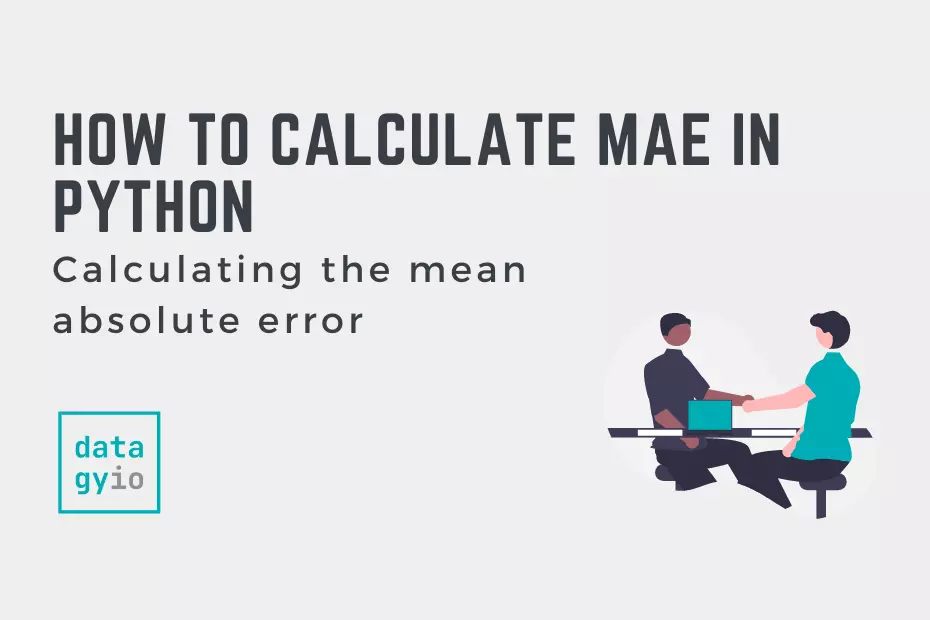# How to Calculate Mean Absolute Error (MAE) in PythonIn this tutorial, you’ll learn how to calculate the mean absolute error, or MAE, in Python. The mean absolute error can help measure the accuracy of a given machine learning model. The MAE can be a good complement or alternative to the mean squared error (MSE).

By the end of this tutorial, you’ll have learned:

• What the mean absolute error is
• How to interpret the mean absolute error
• How to calculate the mae in Python

Let’s get started!

## What is the Mean Absolute Error

The mean absolute error measures the average differences between predicted values and actual values. The formula for the mean absolute error is:

In calculating the mean absolute error, you

1. Find the absolute difference between the predicted value and the actual value,
2. Sum all these values, and
3. Find their average.

This error metric is often used in regression models and can help predict the accuracy of a model.

## How Does the MAE Compare to MSE?

The mean absolute error and the mean squared error are two common measures to evaluate the performance of regression problems. There are a number of key differences betwee the two:

• Unlike the mean squared error (MSE), the MAE calculates the error on the same scale as the data. This means it’s easier to interpret.
• The MAE doesn’t square the differences and is less susceptible to outliers

Both values are negatively-oriented. This means that, while both range from 0 to infinity, lower values are better.

## How do You Interpret the Mean Absolute Error

Interpreting the MAE can be easier than interpreting the MSE. Say that you have a MAE of 10. This means that, on average, the MAE is 10 away from the predicted value.

In any case, the closer the value of the MAE is to 0, the better. That said, the interpretation of the MAE is completely dependent on the data. In some cases, a MAE of 10 can be incredibly good, while in others it can mean that the model is a complete failure.

The interpretation of the MAE depends on:

1. The range of the values,
2. The acceptability of error

For example, in our earlier example of a MAE of 10, if the values ranged from 10,000 to 100,000 a MAE of 10 would be great. However, if the values ranged from 0 through 20, a MAE would be terrible.

The MAE can often be used interpreted a little easier in conjunction with the mean absolute percentage error (MAPE). Calculating these together allows you to see the scope of the error, relative to your data.

## Calculate the Mean Absolute Error in Python

In this section, you’ll learn how to calculate the mean absolute error in Python. In the next section, you’ll learn how to calculate the MAE using sklearn. However, it can be helpful to understand the mechanics of a calculation.

We can define a custom function to calculate the MAE. This is made easier using numpy, which can easily iterate over arrays.

``````# Creating a custom function for MAE
import numpy as np

def mae(y_true, predictions):
y_true, predictions = np.array(y_true), np.array(predictions)
return np.mean(np.abs(y_true - predictions))
``````

Let’s break down what we did here:

1. We imported numpy to make use of its array methods
2. We defined a function `mae`, that takes two arrays (true valuse and predictions)
3. We converted the two arrays into Numpy arrays
4. We calculated the mean of the absolute differences between iterative values in the arrays

Let’s see how we can use this function:

``````# Calculating the MAE with a custom function
import numpy as np

def mae(y_true, predictions):
y_true, predictions = np.array(y_true), np.array(predictions)
return np.mean(np.abs(y_true - predictions))

true = [1,2,3,4,5,6]
predicted = [1,3,4,4,5,9]

print(mae(true, predicted))

# Returns: 0.833``````

We can see that in the example above, a MAE of 0.833 was returned. This means that, on average, the predicted values will be 0.833 units off.

In the following section, you’ll learn how to use sklearn to calculate the MAE.

## Use Sklearn to Calculate the Mean Absolute Error (MAE)

In this section, you’ll learn how to use sklearn to calculate the mean absolute error. Scikit-learn comes with a function for calculating the mean absolute error, `mean_absolute_error`. As with many other metrics, with function is in the `metrics` module.

Let’s see what the function looks like:

``````# Importing the function
from sklearn.metrics import mean_absolute_error

mean_absolute_error(
y_true=,
y_pred=
)``````

The function takes two important parameters, the true values and the predicted values.

Now let’s recreate our earlier example with this function:

``````import numpy as np
from sklearn.metrics import mean_absolute_error

true = [1,2,3,4,5,6]
predicted = [1,3,4,4,5,9]

print(mean_absolute_error(true, predicted))

# Returns: 0.833``````

## Conclusion

In this tutorial, you learned about the mean absolute error in Python. You learned what the mean absolute error, or MAE, is and how it can be interpreted. You then learned how to calculate the MAE from scratch in Python, as well as how to use the Scikit-Learn library to calculate the MAE.